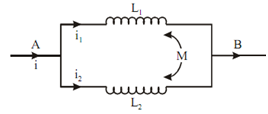## Inductors in Parallel Assignment Help

Assignment Help: >> Inductances in Series and Parallel - Inductors in Parallel

Inductors in Parallel:Figure: Parallel Inductors

Let the two inductors connected in parallel be L1 and L2, and current flowing in them be i1 and i2 respectively. Let the mutual inductance in among the two be M.

Now, i = i1 + i2

Case 1

Whereas the two fields assist each other, then

L =       L1 L2 - M2 / (L1 + L2 - 2M)

Case 2

When the two of the fields oppose each other, then

L =   L1 L2 - M2 / L1 + L2 + 2M